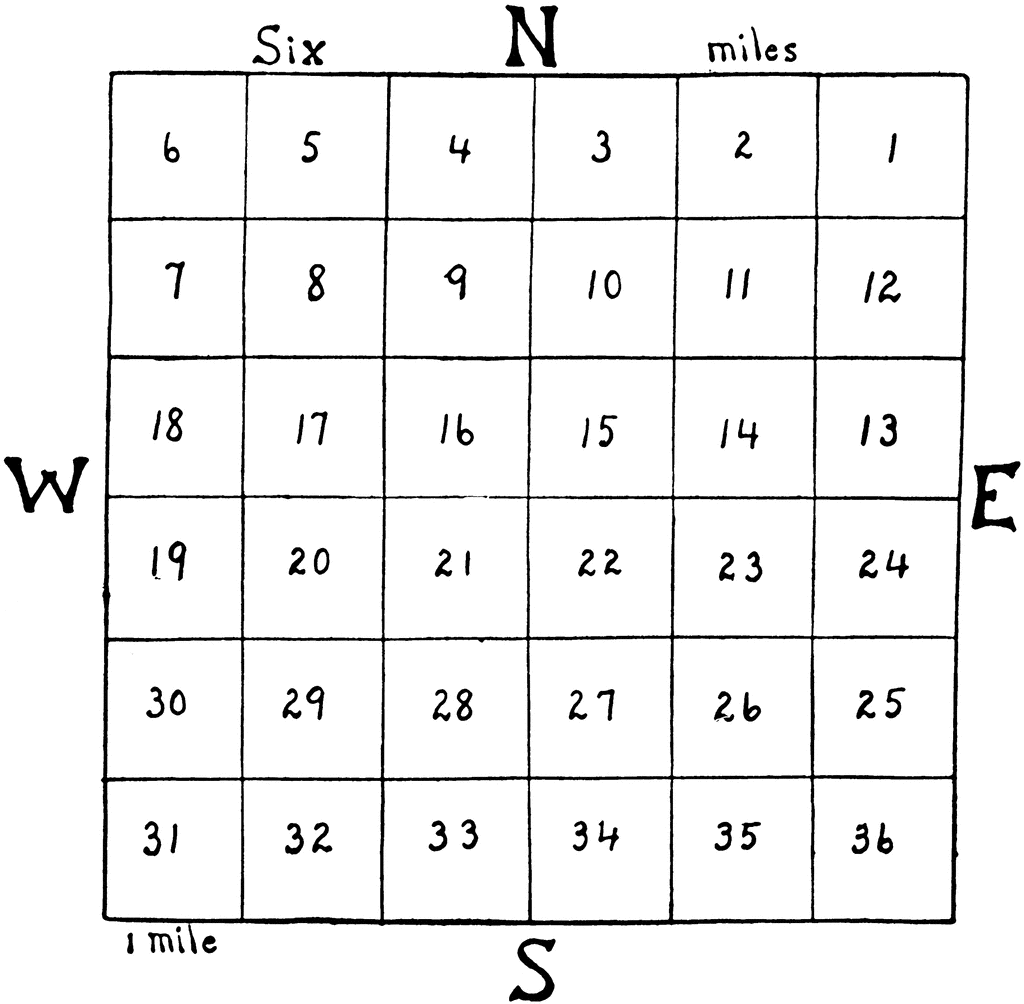Intro text, can be displayed through an additional field

## How Many Acres to a Section

When it comes to land measurements, there are various units used around the world. One such unit is a section, which is commonly used in the United States and Canada. If you're wondering how many acres are in a section, you've come to the right place. In this article, we'll explore the concept of sections, their historical significance, and the exact calculation of how many acres are in a section.

### Understanding Sections

A section is a unit of land measurement that is used primarily in the United States and Canada. It is commonly used to divide large tracts of land, such as townships or ranges, into smaller, more manageable parcels. The concept of sections dates back to the Public Land Survey System (PLSS), which was established in the early 19th century to facilitate the distribution and settlement of public lands.

Each section is a square, measuring approximately one mile on each side. In total, a section consists of 640 acres. This means that there are exactly 640 acres in a section, making it a convenient unit for measuring and dividing large areas of land.

### Calculating Acres in a Section

To calculate the number of acres in a section, you simply multiply the length of one side of the section by itself. Since each side of a section is one mile long, the calculation would be:

1 mile x 1 mile = 1 square mile

Since there are 640 acres in a square mile, the calculation becomes:

1 square mile x 640 acres = 640 acres

Therefore, there are 640 acres in a section.

### FAQs about Acres in a Section

#### Q: Why are sections divided into 640 acres?

A: The division of sections into 640 acres is based on historical factors. The Public Land Survey System was designed to distribute land to settlers in a fair and efficient manner. The 640-acre section size allowed for a balance between providing enough land for agricultural purposes and ensuring manageable parcel sizes for settlement.

#### Q: Can sections be further divided?

A: Yes, sections can be further divided into smaller units. The most common subdivision is a quarter section, which consists of 160 acres. Quarter sections can be further divided into smaller parcels, such as 40-acre plots or 10-acre plots.

#### Q: Are sections used in land measurements outside of the United States and Canada?

A: No, sections are primarily used in the United States and Canada. Other countries may have their own systems of land measurement, such as hectares or square meters.

### Conclusion

In conclusion, a section is a unit of land measurement that consists of 640 acres. This measurement is commonly used in the United States and Canada to divide large tracts of land. The calculation of how many acres are in a section is straightforward, as each side of a section is one mile long. Understanding the concept of sections and their acreage is essential for anyone involved in land surveying, real estate, or agricultural activities.

## Related video of how many acres to a section

Ctrl
Enter
Noticed oshYwhat?
Highlight text and click Ctrl+Enter
We are in
Search and Discover » how many acres to a section
Update Info